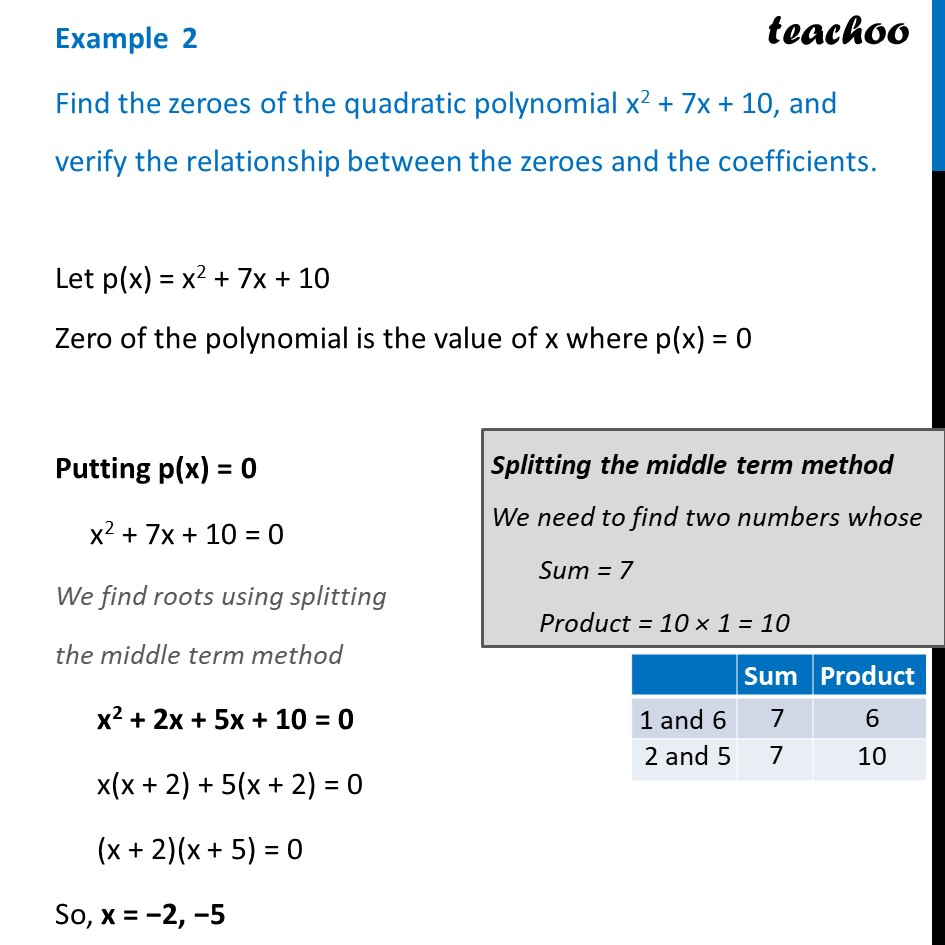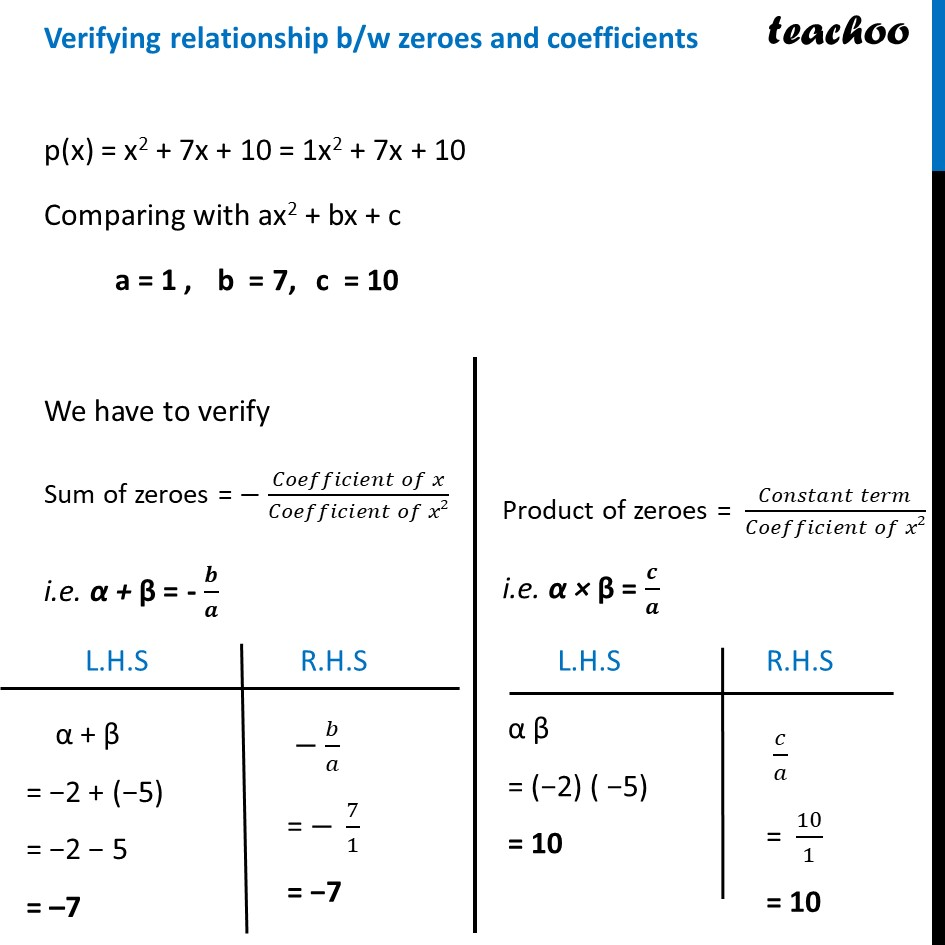Examples

Chapter 2 Class 10 Polynomials
Serial order wiseLearn in your speed, with individual attention - Teachoo Maths 1-on-1 Class

### Transcript

Example 2 Find the zeroes of the quadratic polynomial x2 + 7x + 10, and verify the relationship between the zeroes and the coefficients. Let p(x) = x2 + 7x + 10 Zero of the polynomial is the value of x where p(x) = 0 Putting p(x) = 0 x2 + 7x + 10 = 0 We find roots using splitting the middle term method x2 + 2x + 5x + 10 = 0 x(x + 2) + 5(x + 2) = 0 (x + 2)(x + 5) = 0 So, x = −2, −5 Therefore, α = -2 & β = −5 are the zeroes of polynomial Splitting the middle term method We need to find two numbers whose Sum = 7 Product = 10 × 1 = 10 Verifying relationship b/w zeroes and coefficients p(x) = x2 + 7x + 10 = 1x2 + 7x + 10 Comparing with ax2 + bx + c a = 1 , We have to verify Sum of zeroes = − (𝐶𝑜𝑒𝑓𝑓𝑖𝑐𝑖𝑒𝑛𝑡 𝑜𝑓 𝑥)/(𝐶𝑜𝑒𝑓𝑓𝑖𝑐𝑖𝑒𝑛𝑡 𝑜𝑓 𝑥2) i.e. α + β = - 𝒃/𝒂 Product of zeroes = (𝐶𝑜𝑛𝑠𝑡𝑎𝑛𝑡 𝑡𝑒𝑟𝑚)/(𝐶𝑜𝑒𝑓𝑓𝑖𝑐𝑖𝑒𝑛𝑡 𝑜𝑓 𝑥2) i.e. α × β = 𝒄/𝒂 α + β = −2 + (−5) = −2 − 5 = –7 − 𝑏/𝑎 = − 7/1 = −7 α β = (−2) ( −5) = 10 𝑐/𝑎 = 10/1 = 10 Since, L.H.S = R.H.S Hence relationship between zeroes & coefficient is verified